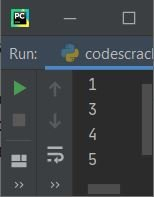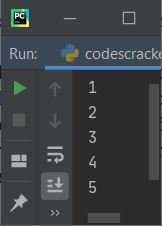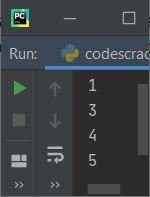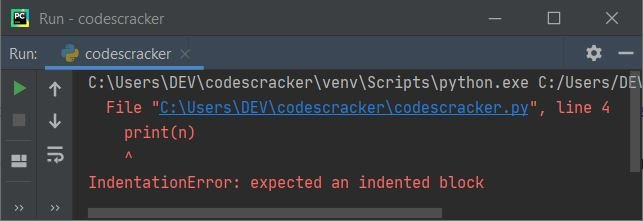# pass statement in Python with examples

As its name suggests, the "pass" statement does nothing, as it gets treated as a null statement. Now, this question may arise in your mind:

• If the "pass" statement does nothing, then why do we need/use it, or why does Python provide this statement?

The answer to this question may depend on the programmer's needs. But I've provided two solid reasons or answers for the above question, namely:

• We can use the "pass" statement wherever the statement is required to avoid syntax errors, and only if that section of the program does not require any action.
• We can also use the "pass" statement if we want to add some blocks of codes in the future. In other words, the "pass" statement can also be used as a placeholder for whatever code we want to write in the future.

Important: The "pass" statement does nothing; it is only required when syntactically needed to avoid syntax errors, but actually does nothing. That is, if the program needs to provide the statement but we want to do nothing, then we can use the "pass" keyword or statement there.

## Syntax of the Python pass statement

The complete statement of "pass" is nothing but the "pass" keyword itself; therefore, if we talk about its syntax, then it is just the keyword "pass," as shown below:

`pass`

We can use the "pass" statement wherever we want in the whole Python program, such as:

• We can use it in a conditional block.
• We can use it in a user-defined function.
• We can also use it in classes.

## Examples of a pass statement

The theory part of the "pass" statement is completed. Therefore, it's time to follow its example. An example helps a lot to understand the topic in the world of computer programming like Python.

```nums = [1, 2, 3, 4, 5]
for n in nums:
if n==2:
pass
else:
print(n)```

The snapshot given below shows the sample output produced by the above example program on the "pass" statement:The number 2 is skipped to print, as shown in the sample output above.Because I've applied the condition "n == 2," whenever the value of n becomes equal to 2, program flow goes inside the body of "if." And inside the body of "if," I've used the "pass" statement, which does nothing.

Since the "pass" statement does nothing, it just passes that block or body where it is present. Let's take another example related to the previous program:

```nums = [1, 2, 3, 4, 5]

for n in nums:
if n==2:
pass
print(n)```

This time, the program produces all five numbers on the output as shown in the snapshot given below:Unlike continue, which forces the loop to continue for its next iteration, skipping the rest statement(s) that lie below the continue keyword in the same indentation causes it to execute. Confused ? Let's take an example to differentiate between these two:

```nums = [1, 2, 3, 4, 5]

for n in nums:
if n==2:
continue
print(n)```

This program produces the following output:Either "pass" works as a placeholder for future code or is used to avoid a syntax error. For example, the following program:

```nums = [1, 2, 3, 4, 5]
for n in nums:
if n == 3:
print(n)```

produces an error like shown in the snapshot given below:Therefore, to avoid these types of syntax errors, we use the "pass" statement like shown in the program given below:

```nums = [1, 2, 3, 4, 5]
for n in nums:
if n == 3:
pass
print(n)```

Python Online Test

« Previous Tutorial Next Tutorial »